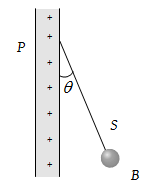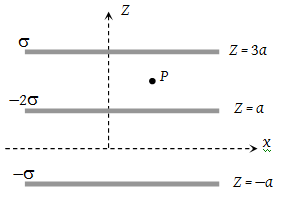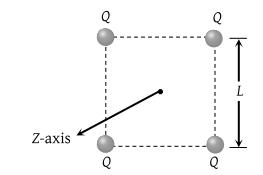Infinite charges of magnitude q each are lying at x =1, 2, 4, 8... meter on X-axis. The value of the intensity of the electric field at point x = 0 due to these charges will be

(1) 12 × 109q N/C

(2) Zero

(3) 6 × 109q N/C

(4) 4 × 109q N/C

Concept Questions :-

Electric field
High Yielding Test Series + Question Bank - NEET 2020

Difficulty Level:

A pendulum bob of mass $30.7×{10}^{-6}\text{\hspace{0.17em}}kg$ and carrying a charge $2×{10}^{-8}\text{\hspace{0.17em}}C$ is at rest in a horizontal uniform electric field of 20000 V/m. The tension in the thread of the pendulum is $\left(g=9.8\text{\hspace{0.17em}}m/{s}^{2}\right)$

(1) $3×{10}^{-4}\text{\hspace{0.17em}}N$

(2) $4×{10}^{-4}\text{\hspace{0.17em}}N$

(3) $5×{10}^{-4}\text{\hspace{0.17em}}N$

(4) $6×{10}^{-4}\text{\hspace{0.17em}}N$

Concept Questions :-

Electric field
High Yielding Test Series + Question Bank - NEET 2020

Difficulty Level:

A charged ball B hangs from a silk thread S, which makes an angle θ with a large charged conducting sheet P, as shown in the figure. The surface charge density σ of the sheet is proportional to(1) sin θ

(2) tan θ

(3) cos θ

(4) cot θ

Concept Questions :-

Electric field
High Yielding Test Series + Question Bank - NEET 2020

Difficulty Level:

Two-point charges +8q and –2q are located at x = 0 and x = L respectively. The location of a point on the x-axis at which the net electric field due to these two point charges is zero is

(1) 8 L

(2) 4 L

(3) 2 L

(4) $\frac{L}{4}$

Concept Questions :-

Electric field
High Yielding Test Series + Question Bank - NEET 2020

Difficulty Level:

Three infinitely long charge sheets are placed as shown in the figure. The electric field at point P is(1) $\frac{2\sigma }{{\epsilon }_{o}}\stackrel{^}{k}$

(2) $-\frac{2\sigma }{{\epsilon }_{o}}\stackrel{^}{k}$

(3) $\frac{4\sigma }{{\epsilon }_{o}}\stackrel{^}{k}$

(4) $-\frac{4\sigma }{{\epsilon }_{o}}\stackrel{^}{k}$

Concept Questions :-

Electric field
High Yielding Test Series + Question Bank - NEET 2020

Difficulty Level:

Two infinitely long parallel conducting plates having surface charge densities +σ and –σ respectively, are separated by a small distance. The medium between the plates is a vacuum. If ε0 is the dielectric permittivity of vacuum, then the electric field in the region between the plates is

(1)

(2) $\frac{\sigma }{2{\epsilon }_{o}}volts/meter$

(3) $\frac{\sigma }{{\epsilon }_{o}}volts/meter$

(4) $\frac{2\sigma }{{\epsilon }_{o}}volts/meter$

Concept Questions :-

Electric field
High Yielding Test Series + Question Bank - NEET 2020

Difficulty Level:

Four-point +ve charges of the same magnitude (Q) are placed at four corners of a rigid square frame as shown in the figure. The plane of the frame is perpendicular to Z-axis. If a –ve point charge is placed at a distance z away from the above frame (z<<L) then(1) – ve charge oscillates along the Z-axis.

(2) It moves away from the frame

(3) It moves slowly towards the frame and stays in the plane of the frame

(4) It passes through the frame only once.

Concept Questions :-

Coulomb's law
High Yielding Test Series + Question Bank - NEET 2020

Difficulty Level:

A cylinder of radius R and length L is placed in a uniform electric field E parallel to the cylinder axis. The total flux for the surface of the cylinder is given by

(1) $2\pi {R}^{2}E$

(2) $\pi {R}^{2}/E$

(3) $\left(\pi {R}^{2}-\pi R\right)/E$

(4) Zero

Concept Questions :-

Gauss law
High Yielding Test Series + Question Bank - NEET 2020

Difficulty Level:

Electric field at a point varies as r° for

(1) An electric dipole

(2) A point charge

(3) A plane infinite sheet of charge

(4) A line charge of infinite length

Concept Questions :-

Electric field
High Yielding Test Series + Question Bank - NEET 2020

Difficulty Level:

An electric charge is placed at the centre of a cube of side α. The electric flux on one of its faces will be

(1) $\frac{q}{6{\epsilon }_{0}}$

(2) $\frac{q}{{\epsilon }_{0}{a}^{2}}$

(3) $\frac{q}{4\pi {\epsilon }_{0}{a}^{2}}$

(4) $\frac{q}{{\epsilon }_{0}}$

Concept Questions :-

Gauss law